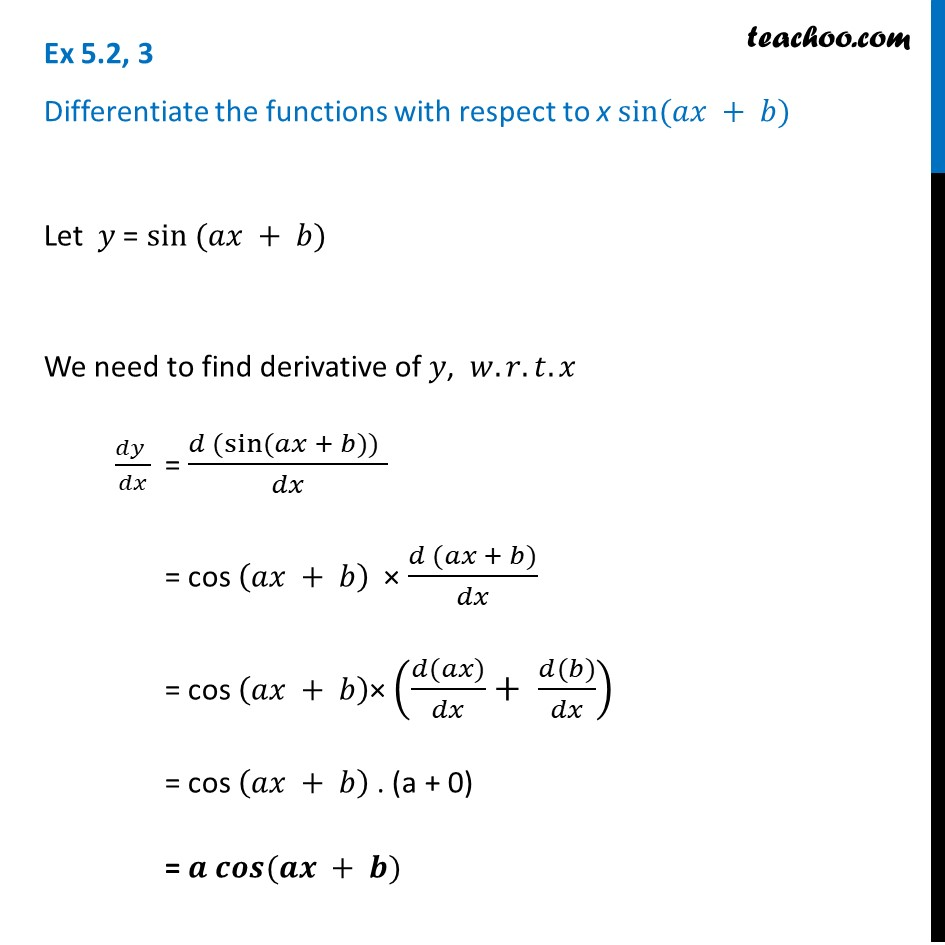Finding derivative of a function by chain rule

Chapter 5 Class 12 Continuity and Differentiability
Concept wiseLearn in your speed, with individual attention - Teachoo Maths 1-on-1 Class

### Transcript

Ex 5.2, 3 Differentiate the functions with respect to x sin⁡(𝑎𝑥 + 𝑏) Let 𝑦 = sin (𝑎𝑥 + 𝑏) We need to find derivative of 𝑦, 𝑤.𝑟.𝑡.𝑥 (𝑑𝑦 )/𝑑𝑥 = (𝑑 (sin⁡〖(𝑎𝑥 + 𝑏)〗)" " )/𝑑𝑥 = cos (𝑎𝑥 + 𝑏) × (𝑑 (𝑎𝑥 + 𝑏))/𝑑𝑥 = cos (𝑎𝑥 + 𝑏)× ((𝑑(𝑎𝑥))/𝑑𝑥+ (𝑑(𝑏))/𝑑𝑥) = cos (𝑎𝑥 + 𝑏) . (a + 0) = 𝒂 𝒄𝒐𝒔⁡(𝒂𝒙 + 𝒃)